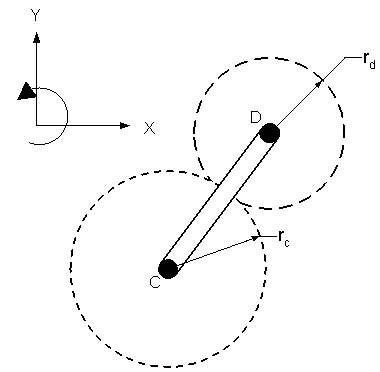# Angular velocity of gears

Gear C has radius 0.12 m and rotates with angular velocity 1.9 k rad/s. The connecting link rotates at angular velocity 1.5 k rad/s. Gear D has radius 0.05 m. Find the angular velocity of gear D (in rad/s). Note that gear C is pinned to ground and gear D is a planetary gear.Since gear c is pinned, the gear ratio ωc/ωd=rd/rc does not directly apply here right? I wonder if the correct solution would be ωd=ωc x rcd / rd. I got -2.125 rad/s. Thanks.

TSny
Homework Helper
Gold Member
Hello.

At the point of contact of the gears, suppose you pick a point ##c## on the rim of gear C and a point ##d## on the rim of gear D as shown.

Let ##\vec{V_c}## and ##\vec{V_d}## be the velocities of those points, respectively, as measured relative to the fixed point at the center of gear C.

How are ##\vec{V_c}## and ##\vec{V_d}## related?

#### Attachments

Last edited:
Vc and Vd are the same

TSny
Homework Helper
Gold Member
OK. So, ##\vec{V_c}## = ##\vec{V_d}##. If you can express each of these velocities in terms of the angular velocities of the gears (##\omega_C## and ##\omega_D##) and the angular velocity of the link (##\omega_L##), then you would have an equation that you could solve for ##\omega_D##.

Start with the velocity vector ##\vec{V_c}##. Suppose you introduce a unit vector ##\hat{t}## that is tangent to the gears at the point of contact, as shown. How can you express ##\vec{V_c}## in terms of ##\omega_C##, ##r_C##, and ##\hat{t}##?

Last edited: